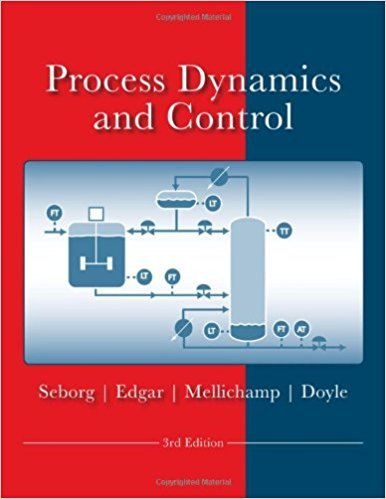×
Get Full Access to Process Dynamics And Control - 3 Edition - Chapter 4 - Problem 4.3
Get Full Access to Process Dynamics And Control - 3 Edition - Chapter 4 - Problem 4.3

×

# The dynamic behavior of a pressure sensor/transmitter canISBN: 9780470128671 148

## Solution for problem 4.3 Chapter 4

Process Dynamics and Control | 3rd Edition

• Textbook Solutions
• 2901 Step-by-step solutions solved by professors and subject experts
• Get 24/7 help from StudySoup virtual teaching assistantsProcess Dynamics and Control | 3rd Edition

4 5 1 383 Reviews
19
3
Problem 4.3

The dynamic behavior of a pressure sensor/transmitter can be expressed as a first-order transfer function (in deviation variables) that relates the measured value P m to the actual pressure, P: P,;,(s) 1 P'(s) 30s + 1 Both P,;, and P' have units of psi and the time constant has units of seconds. Suppose that an alarm will sound if P m exceeds 45 psi. If the process is initially at steady state, and then P suddenly changes from 35 to 50 psi at 1:10PM, at what time will the alarm sound?

Step-by-Step Solution:

Problem 4.3The dynamic behavior of a pressure sensor/transmitter can be expressed as a first-ordertransfer function (in deviation variables) that relates the measured value P m to the actualpressure, P: P m) 1 P (s) = 30s + 1 Both P and P have units of psi and the time constant has units of seconds. Suppose that an malarm will sound if P m exceeds 45 psi. If the process is initially at steady state, and then Psuddenly changes from 35 to 50 psi at 1:10PM, at what time will the alarm sound Step-by-step solution Step 1 of 6 ^Write the transfer function for the pressure transmitter. P ms) 1 P s) = 30s + 1 ……..(1)Where, P ms) is the measured value of pressure. P(s) is the actual value of pressure.The step change from steady state to final state is 35 psi to 50 psi.The actual value of pressure is, P(s) = 15 . 15 sSubstitute s for P(s) in Equation (1). P m) 15 = 1 …..(2) ( s 30s + 1 1 15 Pm(s) = 30s + 1 × 5

Step 2 of 6

Step 3 of 6

##### ISBN: 9780470128671

Unlock Textbook Solution